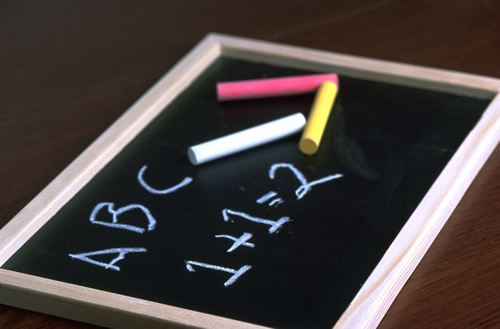• Marvelous Math Strategies1.    Start at the greater number, count up for addition and back for subtraction.
4 + 2 = 6   Say "four. "  Count up 5,  6.
4 - 2 = 2  Say "four. "  Count back 3, 2.

2.  Use a number line or grid.
Don't forget to hop the right direction!  "Subtract go back."

3.  Use "Zero Power."  Anything plus or minus zero equals itself.

4.  Recall sums of ten by using your"hand calculator" ( your ten fingers.)
Four fingers up and six fingers down equals ten.  What other ways make ten?

5.  Sing your "Doubles Rap."  Look for examples of double facts  in your home or environment.

Doubles Rap

It’s the doubles, baby

Let’s go, let’s go…

It’s the doubles, baby

0+ 0= 0 Oh!

1+ 1= 2 Ew!

2+ 2 = 4 More

3 + 3 = 6 Kicks!

4 + 4 = 8 That’s great.

5 + 5 = 10 Again.

6 +6 = 12 Dig and delve.

7 + 7 = 14 Mrs. Sheppard's a queen.

8 + 8 = 16 Jellybean.

9 + 9 = 18 Let’s lean.

10 + 10 = 20 That’s plenty.

6.  Visualize a ten frame.

7.  Memorize doubles plus one facts.
If 6 + 6 = 12, then 6 + 7 = 13
If 8 + 8 = 16, then 8 + 9 = 17

Check out this Council Rock math link.  Great for all  skill levels and mathematical concepts!